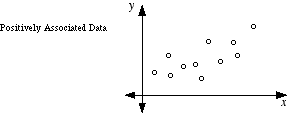index: click on a letter A B C D E F G H I J K L M N O P Q R S T U V W X Y Z A to Z index index: subject areas numbers & symbols sets, logic, proofs geometry algebra trigonometry advanced algebra & pre-calculus calculus advanced topics probability & statistics real world applications multimedia entrieswww.mathwords.com about mathwords website feedback

 Positively Associated Data A relationship in paired data in which the two sets of data tend to increase together or decrease together. In a scatterplot, positively associated data tend to follow a pattern from the lower left to the upper right. Positively associated data have a positive correlation coefficient.See also